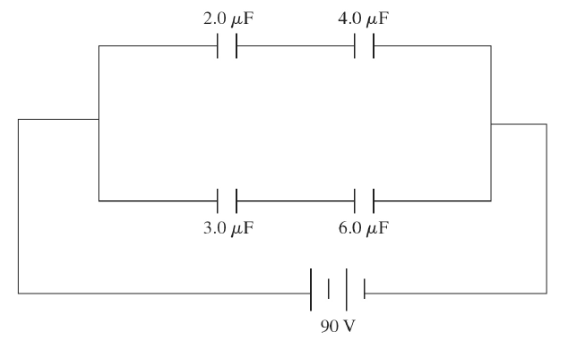# Problem: A system of four capacitors are connected across a 90 -V voltage source as shown in the figure. a) What is the potential difference across the plates of the 6.0 uF capacitor? b) What is the charge on the 3.0 uF capacitor?

###### FREE Expert Solution

Potential difference:

$\overline{){\mathbf{V}}{\mathbf{=}}\frac{\mathbf{Q}}{\mathbf{C}}}$

For two capacitors in series, equivalent capacitance is:

$\overline{){{\mathbf{C}}}_{\mathbf{e}\mathbf{q}}{\mathbf{=}}\frac{{\mathbf{C}}_{\mathbf{1}}{\mathbf{C}}_{\mathbf{2}}}{{\mathbf{C}}_{\mathbf{1}}\mathbf{+}{\mathbf{C}}_{\mathbf{2}}}}$

Charge:

$\overline{){\mathbf{Q}}{\mathbf{=}}{\mathbf{C}}{\mathbf{V}}}$

82% (432 ratings)###### Problem Details

A system of four capacitors are connected across a 90 -V voltage source as shown in the figure.

a) What is the potential difference across the plates of the 6.0 uF capacitor?

b) What is the charge on the 3.0 uF capacitor?